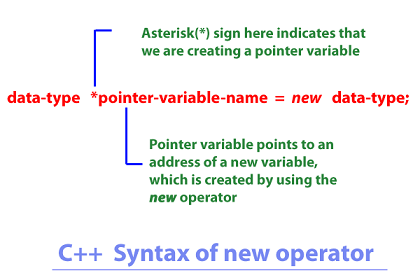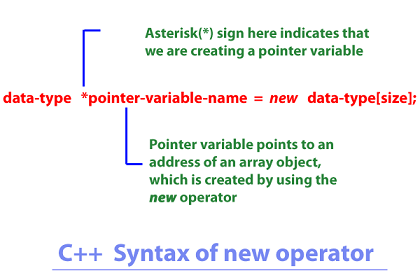< Prev
Next >

# C++ new Operator

The C++ language provides us two memory management operators, such as: new and delete -
• The new operator allows us to allocate the memory space for the creation of a new variable or a new object at the runtime.
• The delete operator allows us to free up the memory space which was allocated at the runtime, for the creation of a new variable or an new object.
In this tutorial, we are going to discuss the new memory management operator.

• ## new operator

• The new memory management operator allows us to create a new variable or a new object by allocating it an appropriate memory space at the runtime(dynamically).

There are two syntax of using new memory management operator depending on what you want to create i.e. if you are creating a variable or an object.

## new operator creating a variable

The new memory management operator allows us to not only create a new variable by allocating it an appropriate memory space at the runtime(dynamically), but also allows us to initialize the variable at the time of its creation.

Let us see the syntax of how to use the new operator to allocate the memory space for a new variable.Understanding the syntax of new operator to create a variable:
• The pointer-variable-name is the name of pointer variable which points to the memory address of a newly created variable.
• The new operator is used to allocate an appropriate memory space to the variable of a specified data-type.
• The data-type is the data-type used to create its new variable by allocating it an appropriate memory space.

``````//C++ Example of new operator to create a variable

#include<iostream>

using namespace std;

int main()
{
//Creating an int variable by using the new operator
int *p = new int;

//Initializing the newly created int variable
*p = 10;
cout<< "The int value at the address pointed by pointer variable : " << *p << "\n";
cout<< "The memory address allocated to pointer variable : " << p;

//Creating and initializing a float variable using the new operator in a single line
float *f = new float(10.5);

cout<< "The float value at the address pointed by pointer variable : " << *f << "\n";
cout<< "The memory address allocated to pointer variable : " << f;

return 0;
}``````

## Output

``````The int value at the address pointed by pointer variable : 10
The memory address allocated to pointer variable : 0x6f1690
The float value at the address pointed by pointer variable : 10.5
The memory address allocated to pointer variable : 0x6f0f48``````

Now look at the following part from our program:
``````//Creating and initializing a float variable using new operator in a single line
float *f = new float(10.5);``````

This line of code has used the new operator to not only create a float variable but also to initialize it with a value within a single line.

• ## new operator creating an object(an array object)

• Let us see the syntax of how to use the new operator to allocate the memory space for the creation of a new object i.e. an array object.Understanding the syntax of new operator to create an array object:
• The pointer-variable-name is the name of pointer variable which points to the memory address of a newly created array object.
• The new operator is used to allocate an appropriate memory space to the array object of the specified data-type.
• The data-type is the data-type used to create an object by allocating it an appropriate memory space.
• The size specifies the number of elements in an array, that are going to be allocated the memory space.

``````//C++ Example of new operator to create an array object

#include<iostream>

using namespace std;

int main()
{

//Creating a memory space for a char array with 5 elements, using new operator
char *arr = new char;

arr = 'a';
arr = 'b';
arr = 'c';
arr = 'd';
arr = 'e';

cout<< "The multiple char values in the char array: \n";

for(int i=0;i<5;i++)
{
cout<< arr[i] << "\n";
}

cout<< "The memory address allocated to pointer variable : " << arr;

return 0;
}``````

## Output

``````The multiple char values in the char array:
a
b
c
d
e
The memory address allocated to pointer variable : abcde``````

In the next tutorial, we are going to explain the delete memory management operator, so please stay tuned.